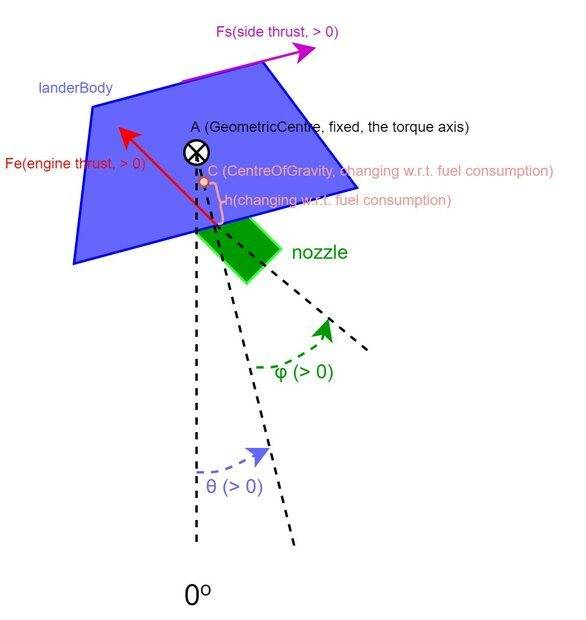# Solving Sub-Problem for OpenAI Lunar Lander v2: Seeking Advice

• I
• genxium

#### genxium

I'm currently working on a pet project which is similar to the OpenAI Lunar Lander v2 (hence the problem is in a 2D context), and seeking help for a sub-problem that's been blocking me for a while.

At any instant of time, I'm to find
• Fe: magnitude of main engine thrust, must be >0
• Fs: magnitude of side engine thrust (>0 to point to the "right w.r.t. lander body", and < 0 to point to opposite direction),
• φ: tilted angle of main engine nozzle w.r.t. lander body, should be within [-π/2, +π/2]

, given
• ax: the expected x-acceleration of COG (center of gravity, same below)
• ay: the expected y-acceleration of COG
• βC: the expected angular acceleration w.r.t axis through COG and perpendicular to the plane (this screen)
• m: current mass of the whole lander
• IC: current moment of inertia of the whole lander w.r.t. same axis of βC
• θ: current tilted angle of the lander body w.r.t. the fixed gravity direction
• h: current distance of COG and the nozzle hinge as shown in the figure below
• g: fixed gravity acceleration
• H: total height of the lander bodyTo my understanding, the dynamics are (don't know whether I can use TeX here, tried wrapping with  and ## but preview didn't work)
• -Fe*sin(θ+φ) + Fs*cosθ = ax*m
• Fe*cos(θ+φ) + Fs*sinθ - g*m = ay*m
• Fe*sinφ*h + Fs*(H-h) = βC*IC
and the "to find variables (in red)" seem non-trivial to solve for.

Is there any advice for solving them at least numerically? An analytical solution will certainly be much appreciated but not a pursuit here.

Last edited: